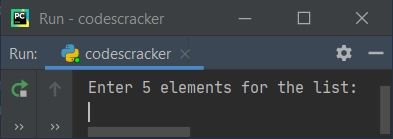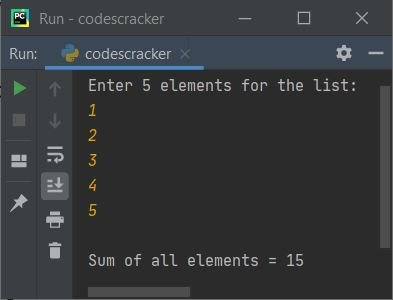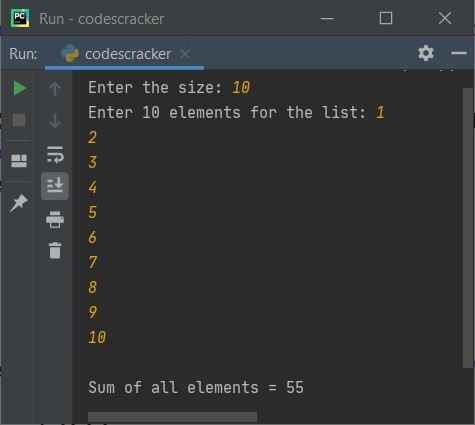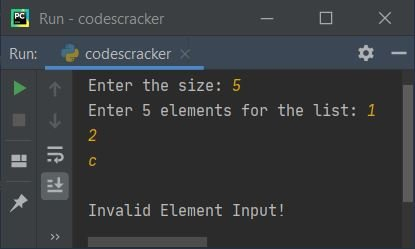# Python Program to Find Sum of all Elements in a List

• Find and print sum of all elements in a list of 5 elements
• Find and print sum of all elements in a list of n elements

## Find Sum of all Elements in a List

The question is, write a python program to print sum of all elements in a list. The program given below is answer to this question:

```nums = []
print("Enter 5 elements for the list: ")
for i in range(5):
val = int(input())
nums.append(val)

sum = 0
for i in range(5):
sum = sum + nums[i]

print("\nSum of all elements =", sum)```

Here is its sample run:Now provide the input say 1, 2, 3, 4, 5 as five elements for the list and press `ENTER` key to find and print the sum of all these 5 elements (numbers) like shown in the snapshot given below:## Print Sum of all Elements in a List of n Elements

This is basically a modified version of previous program. This program allows user to define the size of list before providing elements to it. In this program, we've applied the addition operation while receiving the element input for the list, to avoid unwanted multiple for loops in the program.

```nums = list()
sum = 0
print("Enter the size: ", end="")
tot = int(input())
print("Enter", tot, "elements for the list: ", end="")
for i in range(tot):
nums.append(int(input()))
sum = sum + nums[i]
print("\nSum of all elements =", sum)```

Here is its sample output after providing the input, 10 as size and 1, 2, 3, 4, 5, 6, 7, 8, 9, 10 as ten elements or numbers:#### Modified Version of Previous Program

This program is created to provide a message to user, when they enters an invalid input. Using try-catch block, we've done the task.

```print("Enter the size: ", end="")
try:
tot = int(input())
print("Enter", tot, "elements for the list: ", end="")
nums = list()
sum = 0
for i in range(tot):
try:
val = int(input())
nums.append(val)
sum = sum + nums[i]
except ValueError:
print("\nInvalid Element Input!")
exit()
print("\nSum of all elements =", sum)
except ValueError:
print("\nInvalid Size Input!")```

This program produces the same/similar output as of previous program, if you provide the exactly same or correct input values. Otherwise if you provide any invalid input like invalid elements input, then the program produces an error like shown in the snapshot given below:Since c not a number, rather it is a character, therefore the program produces an error like shown in above snapshot.

Python Online Test

« Previous Program Next Program »